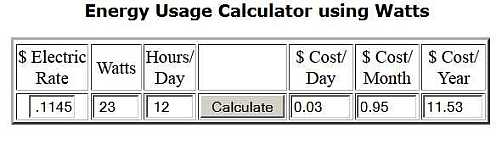﻿ Energy Usage Calculators - Electrical 101# Energy Usage Calculators

## Energy Usage Calculator Example

How much would it cost to have a 23 watt bulb on for 12 hours a day using a \$0.1145 kWh rate?

1. Enter electric rate for using \$0.1145 kWh rate     .1145
2. Enter watts of CFL                                             23
3. Enter number of hours of operation per day         12
4. The resultant cost in \$                                       .03/day, .95/month, 11.53/year

Below are energy calculators you can use to determine approximate cost of operating appliances and electronics. You can calculate daily, monthly, and yearly cost whether they are on, off, or in idle mode. With these calculators you can:

• Convert amps to watts and watts to amps.
• Determine how much it costs to leave a light on for a certain amount of time.
• Compare the cost of an incandescent, CFL, or LED over time.
• Determine cost of phantom power usage (power is turned off).

See Energy Usage Chart for examples of energy usage of appliances and electronics when on, off, or in standby mode.Example

Enter a number in either the amps or watts boxes.

\$ Electric
Rate
Watts
Hours/
Day

\$ Cost/
Day
\$ Cost/
Month
\$ Cost/
Year
\$ Electric
Rate
Amps
Hours/
Day

\$ Cost/
Day
\$ Cost/
Month
\$ Cost/
Year

### Energy Usage Calculator using Amps

When using these calculators, make sure JavaScript is activated.

120V Power Converter

Watts
equals
Amps

240V Power Converter

Watts
equals
AmpsSave Energy

Standby (Phantom) Power

Energy Usage Calculators

Electric Meters

Residential Electric Rates